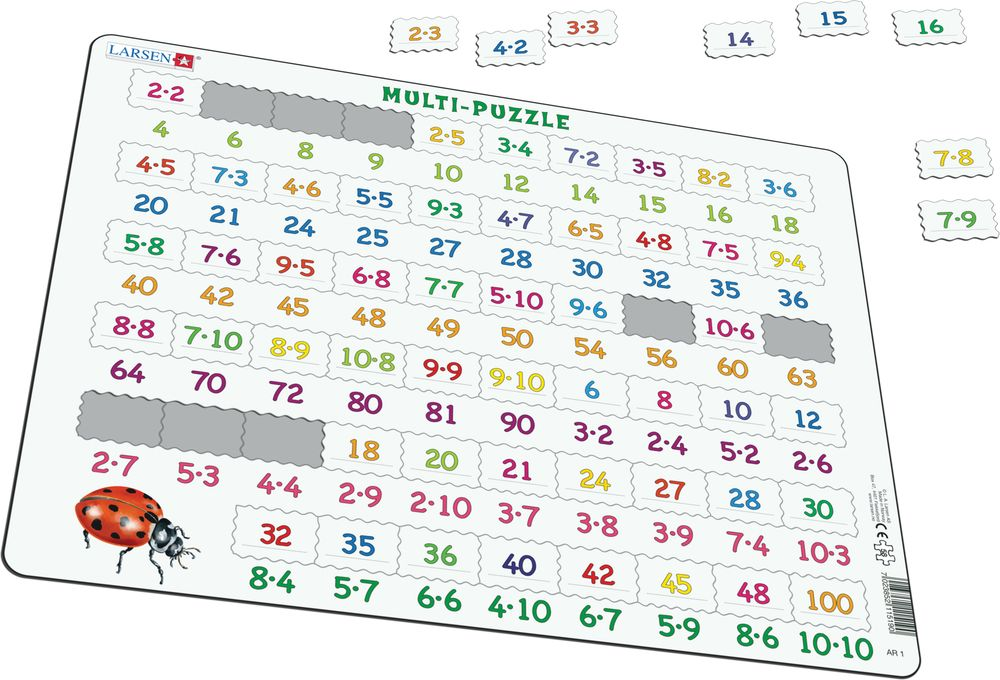# AR1 - Math Puzzle: Multiplication

## Maxi (36.5x28.5 cm) with 58 pieces

•1 x 2 is 2, and 2 x 2 is 4, solve the problem, place the piece, and multiply some more. 2 x 3 is 6, and 2 x 5 is 10 add some more and multiply, no need to borrow or lend! Have some 'maths' fun with this educational multiplication puzzle. In this puzzle, you will find 36 'problems' imprinted on each puzzle piece. The answers are imprinted on the frame, directly underneath. Each number and problem are printed in bright, neon colours to grab the puzzlers' attention. A large spotted ladybird illustration is placed on the bottom left hand of the frame. The puzzle comes complete with 58 curved edged pieces. On the bottom half of the puzzle, you will find the remaining 22 pieces with the answers or numbers on them, which relate to the 'problem' printed on the frame. Each puzzle piece is custom cut to fit the correct place in the tray. This puzzle is best suited for slightly older children, learning to multiply.

AR1 - Math Puzzle: Multiplication is available in the following versions:

• Neutral (EAN/GTIN: 7023852115190)